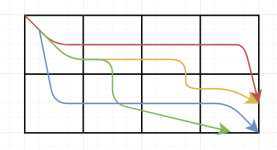# Count the number of ways to traverse a Matrix in C++

Given a 2D matrix with dimensions row X col. The goal is to count the number of ways one can traverse the matrix from cell 0,0 to cell row, col using only right and down moves, i.e. first move can be 0,0 to 0,1 (down) or 1,0 (right) and not 1,1(diagonal).

For Example

## Input

col = 2; row = 4

## Output

Count of number of ways to traverse a Matrix are: 4

## Explanation

The ways in which we can reach from cell 0,0 to 2,4 is shown −## Input

col = 4; row = 3

## Output

Count of number of ways to traverse a Matrix are: 10

## Explanation

We will reduce the problem into smaller recursions. Count ways for
col=3, row=2, then for col=2, row=1 ….. For col=1 or row=1 the answer will be 1. (only right or only down move).

Approach used in the below program is as follows

In this approach we will use a recursion method. At the end for either row or col as 1. There is only one way which is 1 straight right move or 1 straight down move. This will be the terminating condition for recursion.

• Take integers row, col for dimensions of the matrix.

• Function ways_traverse_matrix(int row, int col) takes dimensions and returns the count of the number of ways to traverse a Matrix.

• For row==1, return 1.

• For col==1, return 1.

• Else calculate ways using recursion ways_traverse_matrix(temp_1, col) + ways_traverse_matrix(row, temp_2).

• Here temp_1=previous row number and temp_2=previous column number.

• At the end we will get a count of total ways.

## Example

Live Demo

#include <bits/stdc++.h>
using namespace std;
int ways_traverse_matrix(int row, int col){
if (row == 1){
return 1;
}
else if(col == 1){
return 1;
} else {
int temp_1 = row − 1;
int temp_2 = col − 1;
return ways_traverse_matrix(temp_1, col) + ways_traverse_matrix(row, temp_2);
}
}
int main(){
int col = 2;
int row = 2;
cout<<"Count the number of ways to traverse a Matrix are: "<<ways_traverse_matrix(row, col);
return 0;
}

## Output

If we run the above code it will generate the following output −

Count the number of ways to traverse a Matrix are: 2